# Construction and Application of a Financial Big Data Analysis Model Based on Machine Learning

Construction and Application of a Financial Big Data Analysis Model Based on Machine Learning
School of Computer and Information Engineering, Hebei Finance University, Baoding 071051, China

College of Engineering and Technology, Baoding University of Technology, Baoding 071051, China

Corresponding Author Email:
pangling@hbfu.edu.cn
Page:
345-350
|
DOI:
https://doi.org/10.18280/ria.340313
10 December 2019
|
Accepted:
5 April 2020
|
Published:
30 June 2020
| Citation

OPEN ACCESS

Abstract:

This paper attempts to improve the real-timeliness and value of financial services, and realize scientific decision-making in financial processes. For these purposes, the application mechanism of big data technology in the financial field was discussed in details, and the procedure of financial data preprocessing was explained. Based on user portrait of financial behaviors and machine learning (ML), the authors established a financial big data analysis (BDA) model, in which weight coefficients are assigned reasonably to three networks, namely, Bayesian network (BN), backpropagation neural network (BPNN), and autoregressive integrated moving average (ARIMA) network. In this way, the proposed model combines the merits of all three networks. Experimental results show that the proposed user portrait greatly facilitates the construction of causality in a large sample set, and the prediction curve of the proposed combinatory prediction model achieves the smallest prediction error.

Keywords:

machine learning (ML), financial big data, big data analysis (BDA) model, combinatory prediction

1. Introduction

With the rapid development of Internet technology, recent years has seen the proliferation of big data analysis (BDA) in various industries [1-7]. For example, Google monitors influenza epidemics based on user search records. Taobao assigns credit values to its users by analyzing their data on browsing and purchases.

In the financial field, the application of BDA greatly promotes the update of financial marketing models, bringing shocks as well as immeasurable profits and values to the financial market [8-11]. The accumulation of massive financial data, coupled with the development of BDA technology, has made it possible to perform precision marketing through feature analysis of financial behaviors [12-16].

The financial data are extremely massive and complex, highlighting the importance of BDA models. To speed up computation and shorten computing cycle, many effective computing methods and analysis strategies have been developed by experts at home and abroad. For example, Cummins et al.  combined big data with cloud computing, puts forward the concept of financial big data processing based on cloud platform, and emphasizes that the BDA of financial services should solve the problem of poor timeliness. French and Kelly  designed the topology of a BDA system for financial services: the data resource to be processed are pre-processed in parallel, optimized through clustering, and reasonably allocated to computing nodes; then, the sequence of node calculation is optimized to ensure the real-timeliness and efficiency of data processing. Based on distributed machine learning (ML), Kuzilek et al.  developed a heuristic BDA tool for massive big data streams, and proved that the tool has a good ability to process and analyze big data. Robinson et al.  explained application mechanism of BDA in Internet financial marketing, and compared semantic-based processing of financial big data with multiple time series analysis on financial data.

In the financial industry, the BDA mainly aims to ensure the timeliness and value of financial services. This calls for more in-depth research into data analysis models and relevant algorithms [21-25]. This paper discusses the application mechanism of big data technology in the financial field, gives methods and strategies for preprocessing financial data, builds user portraits based on financial big data, and proposes an ML-based financial BDA model. In the proposed model, weight coefficients are assigned appropriately to Bayesian network (BN), backpropagation neural network (BPNN), and autoregressive integrated moving average (ARIMA) network.

2. Application Mechanism of Big Data Technology in the Financial Field

Suitable storage and processing of big data are the prerequisites for reasonable analysis and application of massive data in the financial field. That is, the massive, disorderly and messy raw financial data should be transformed into modular, orderly and concise data, and the connections between data must be clarified.

Being the earliest architecture of distributed system, the Hadoop Distributed File System (HDFS) enables ordinary computers to store massive data. In the Hadoop cluster, MapReduce is the processing framework for distributed processing of vast data. Based on mapping and protocol, MapReduce converts and aggregates the stored data multiple times, such that programmers, incapable of distributed parallel programming, could run their programs on distributed systems. However, the shuffle mechanism may cause the lost of many input and output ports, resulting in low efficiency.

The Spark framework offers a computationally efficient alternative to scenarios that require iterations (e.g. data mining and the ML). Based on memory computing, the Spark framework can save the intermediate output results of the job in the memory, eliminating the need to read and write HDFS.

The application of the above big data techniques has promoted the innovation of informatized financial marketing models. To realize precision marketing, it is necessary to analyze and mine user behavior data (e.g. financial platform transaction information, e-commerce shopping information, life service payment information, social platform information, bank card and credit card information, and third-party payment information), and build a user portrait system with precise orientation, target and positioning on personalized communication service system. The application process of big data technology in financial marketing is illustrated in Figure 1.

## 1.jpg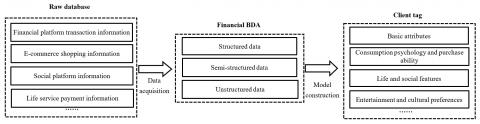Figure 1. The application process of big data technology in financial marketing

3. Preprocessing of Financial Data

The user portrait can be depicted clearly through cluster analysis and model training of the basic user information and financial-related behavior information in the operator data. To screen the information of users frequently engaged in financial activities, this paper focuses on the most popular apps on financial and wealth management, especially stocks and insurance. Through simulated logins, the request-behavior mapping of the relevant financial behavior rules was captured from the apps, and used to create a financial behavior rule database.

In addition, the distributed crawler technology was adopted to capture the code of financial products, facilitating the analysis on the relationship between user attention of financial products and user financial behaviors and that on the correlation between user attributes and different dimensions of their behaviors.

To ensure the accurate association of training set features, the Internet information and rule configuration table were prescreened from the operator dataset based on the main domain name in the Hive Cli mode, and the valid time range of analysis was determined, before the association of valuable financial data. Figure 2 describes the workflow of financial data preprocessing.

The preprocessing of financial data integrates the basic information, income, financial behaviors, interested financial products and other features of each user. Table 1 details the preprocessed data of a user in the training set.

## 2.jpg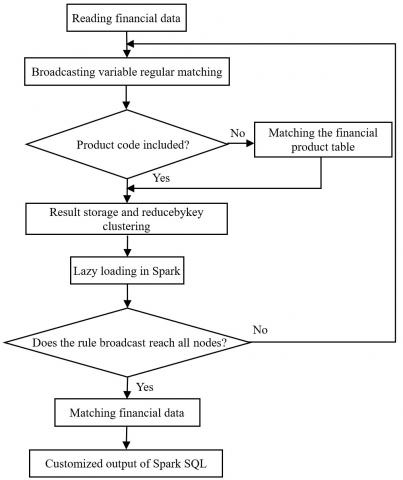Figure 2. The workflow of financial data preprocessing

Table 1. The preprocessed data of a user in the training set

 Item Phone number Age Gender Province City Content 1519034**** 33 Male Jiangsu Xuzhou Item Type of city Phone brand Phone model Phone price Lending platform Content Prefectural city Huawei P30 4,000 Renrendai Item Loan product Loan amount Bank Card type Card Content Xinjihua RMB 50,000 yuan Bank of China Wenhuitong Co-branded credit card
4. User Portrait and Financial BDA Algorithm

In the advertising industry, precision marketing is an effective method to attract consumers. To realize precision marketing, it is necessary to perform financial BDA on the consumption behaviors of users, and generate the feature tags for each user; then, the user portrait could be drawn based on these tags, laying the basis for targeted marketing. The information of feature tags mainly belongs to five dimensions: name, problem domain, time, behavior triggering object, and location. Since the appearance of feature labels is random, this paper proposes a financial BDA model based on probabilistic user portrait and the ML. In the model, weight coefficients are assigned appropriately to BN, BPNN and ARIMA network.

4.1 ML-based financial BDA model

To accurately detail the features of financial behaviors, it is assumed that a user portrait is UP=<L, O, c>, where L=<Li, ci>|i=1, 2, ...n (ci is the probability for the i-th tag to appear; n is the total number of tags), and O＝{<Oj, cj>|j=1, 2, ...m, cj (cj is the probability for the j-th operator to appear; n is the total number of operators). Then, the user portrait can be modelled as:

$C=\underset{i}{\overset{n}{\mathop{\prod }}}\,\left[ c\left( {{L}_{i}}|l\left( {{L}_{i}} \right) \right)\cdot c\left( {{L}_{i}}|O\left( {{L}_{i}} \right) \right) \right]$  (1)

where, l(Li) and O(Li) are the set of left operands and the set of left operators of feature tag Li, respectively. Let P (A|B) be the probability of event A occurring based on event B. Then, the probability of event B can be expressed as:

$P\left( {{b}_{i}}|A \right)=\frac{P\left( {{b}_{i}} \right)P\left( A|{{b}_{i}} \right)}{\underset{i=1}{\overset{m}{\mathop \sum }}\,P\left( {{b}_{i}} \right)P\left( A|{{b}_{i}} \right)}$  (2)

where, ai and bi are the divisions of events A and B, respectively. Any of a1, a2.., an could cause the occurrence of B. The probabilities of small events b1, b2..., bm add up to the probability of event B. Then, the BN was improved through the steps in Figure 3, based on the model of a known user portrait UP.

## 3.jpg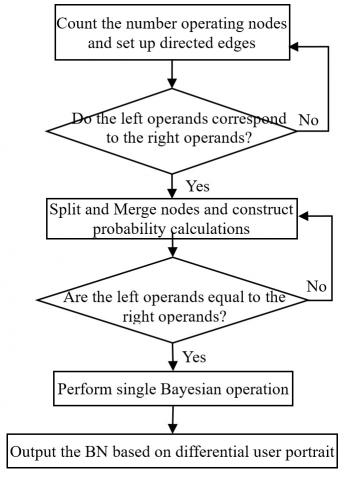Figure 3. The workflow of improving the BN

The original BPNN contains n input layer nodes, t hidden layer nodes, and m output layer nodes. The inputs, actual outputs, and desired outputs of the BPNN are a1, a2…, an, b1, b2..., bm, and c1, c2…, cm, respectively. Let p be the connection weight between nodes. Then, the connection weight between input and hidden layers, and that between hidden and output layers can be respectively denoted as pih and pho. The initial weights of the BPNN are random. Here, the original BPNN is updated by:

\begin{align} & AC{{T}_{i}}=\sum\limits_{h=0}^{t}{{{p}_{ih}}{{a}_{i}}} \\ & {{d}_{i}}={{f}_{sig}}\left( AC{{T}_{i}} \right)=\frac{1}{1+\text{exp}\text{-}AC{{T}_{i}}} \\ & {{c}_{o}}={{f}_{line}}\left( \sum\limits_{i=0}^{n}{{{p}_{ho}}{{d}_{i}}} \right) \\\end{align}  (3)

where, ACTi is the value of the i-th activation node; di is the output of the hidden layer; fsig is the activation function (Sigmoid); fline is the linear function.

Considering the multi-source of financial data, this paper expands the fuzzy rules in the original hidden layer, making the model more adaptable to the nonlinear mapping between financial data. Let Xi and Yi be fuzzy sets, and DXi and DYi be membership functions. Then, the membership function of the input layer can be established as:

$\begin{matrix} \begin{matrix} {{p}_{1-i}}={{D}_{Xi}}\left( {{a}_{i}} \right) & \text{or} \\\end{matrix} & {{p}_{1-i}}={{D}_{Yi}}\left( {{a}_{i}} \right) \\\end{matrix}$  (4)

To characterize the credibility of the fuzzy rules, the first layer of the hidden layer was designed as the multiplication of the input signals. Then, the applicability of the fuzzy rules can be outputted as:

${{p}_{2-i}}=\prod\limits_{i=1}^{n}{{{D}_{{{X}_{i}}}}\left( {{a}_{i}} \right)\cdot {{D}_{{{Y}_{i}}}}\left( {{a}_{i}} \right)}$  (5)

The second layer of the hidden layer normalizes the applicability of fuzzy rules. The ratio of the normalized applicability pk of the k-th rule to the total normalized applicability of all rules can be computed by:

${{p}_{3-i}}={{\overline{q}}_{i}}={{q}_{i}}/\underset{i=0}{\overset{n}{\mathop \sum }}\,({{q}_{i}})$  (6)

The third layer of the hidden layer calculates the value of each fuzzy rule by:

${{p}_{4-i}}=\overline{{{q}_{i}}}{{W}_{i}}=\left( {{e}_{1}}{{a}_{1}}+{{e}_{2}}{{a}_{2}}+\cdots +{{e}_{n}}{{a}_{n}} \right)$  (7)

where, e1, e2, …, en are the consequent parameters.

Then, the output layer summarizes and outputs the signals outputted from the hidden layer:

${{p}_{5-i}}=\underset{i=1}{\overset{n}{\mathop \sum }}\,\left( \overline{{{q}_{i}}}W \right)$  (8)

Based on autoregressive, integral and moving average parameters, the ARIMA network in our model predicts financial information by constructing the linear relationship between past observations and random errors:

${{\eta }_{u}}\left( B \right){{\phi }_{v}}\left( {{B}^{c}} \right){{\nabla }^{d}}\nabla _{C}^{d}\tau ={{\delta }_{w}}\left( B \right){{\Phi }_{W}}\left( {{B}^{C}} \right){{w}_{n}}$  (9)

where, u is the nonfinancial cycle autoregressive term; ηu is the u-order nonfinancial cycle autoregressive function; v is the financial cycle autoregressive term; φv is the v-order financial cycle autoregressive function; C is the time length of the financial cycle; ▽d and ▽CD are the differential functions of nonfinancial and financial cycles, respectively; δw and ΦW are the w-order moving average functions of nonfinancial and financial cycles, respectively; τ is the time point of financial activity; wn is the white noise parameter.

The above-mentioned ARIMA financial BDA model can be constructed in the following steps: First, identify the cyclical factors of financial activities; then, verify the time series of financial activities through the evaluation of financial cycle stability; after that, calculate the estimated values of all parameters, creating a preliminary time series model based on financial BDA.

4.2 Combinatory prediction strategy of financial BDA model

Let a1, a2.., an be the data on actual financial activities, b1t, b2t, ..., bnt be the predicted results at time t, and wBayesian, wBP and wARIMA be the weight coefficients assigned to BN, BPNN, and ARIMA network, respectively. Then, the combinatory prediction result can be expressed as:

$\begin{matrix} {{{\hat{A}}}_{i}}=\sum\limits_{i=1}^{n}{{{w}_{Bayesian}}{{b}_{it}}}+\sum\limits_{i=1}^{n}{{{w}_{BP}}{{b}_{it}}}+\sum\limits_{i=1}^{n}{{{w}_{ARIMA}}{{b}_{it}}} \\ {{w}_{Bayesian}}+{{w}_{BP}}+{{w}_{ARIMA}}=1 \\\end{matrix}$  (10)

The prediction error can be expressed as:

\begin{align} & {{e}_{t}}=\sum\limits_{i=1}^{n}{{{w}_{Bayesian}}\left( {{a}_{i}}-{{b}_{it}} \right)} \\ & +\sum\limits_{i=1}^{n}{{{w}_{BP}}\left( {{a}_{i}}-{{b}_{it}} \right)}+\sum\limits_{i=1}^{n}{{{w}_{ARIMA}}\left( {{a}_{i}}-{{b}_{it}} \right)} \\ & =\sum\limits_{i=1}^{n}{{{w}_{Bayesian}}{{e}_{it}}}+\sum\limits_{i=1}^{n}{{{w}_{BP}}{{e}_{it}}}+\sum\limits_{i=1}^{n}{{{w}_{ARIMA}}{{e}_{it}}} \\\end{align}  (11)

4.3 Calculation of dissimilarity in financial big data and optimization of the ML module

If the data are excessively high-dimensional, it is impossible to process them quickly by traditional clustering algorithms. To optimize the financial BDA model, the first step is to compute the dissimilarity between data clusters, i.e. to perform unified calculation and processing of multi- or high-dimensional data.

Let z1, z2.., zn be n-dimensional datasets on actual financial activities. Then, the continuous nature dissimilarly between the r-th data of dataset zi and the r-th data of dataset zj can be expressed as:

$DI{{S}_{1}}\left( r \right)=\frac{\left| {{z}_{ir}}-{{z}_{jr}} \right|}{Ma{{x}_{r}}-Mi{{n}_{r}}}$  (12)

where, zir is the eigenvalue of the r-th data of dataset zi; Maxr and Minr are the maximum and minimum values of the r-th data in all the n-dimensional datasets, respectively. Then, the dissimilarity of ordinal attributes can be expressed as:

$DI{{S}_{2}}\left( r \right)=\frac{\left| {{z}_{ir}}-{{z}_{jr}} \right|}{{{A}_{r}}-1}$  (13)

where, Ar is the maximums of r in ordered sequence sets 1, 2.., A. The values of the binary and sub-type data attributes solely depend on their types or states, instead of magnitudes. Hence, the dissimilarity of binary and sub-type attributes can be computed by:

$DI{{S}_{3}}\left( r \right)=\left\{ \begin{matrix} 0,{{z}_{ir}}\ne {{z}_{jr}} \\ 1,{{z}_{ir}}={{z}_{jr}} \\\end{matrix} \right.$  (14)

In traditional clustering algorithms, the training module is selected randomly, making the analysis results unstable. The instability can be solved by optimized ML. First, t ML modules were selected according to the applicability of the above rules, and pre-stored as the center points of the BDA. The distance between datasets zi and zj can be expressed as:

$D\left( {{z}_{i}},{{z}_{j}} \right)=\sqrt{{{\left( {{z}_{i1}},{{z}_{j1}} \right)}^{2}}+{{\left( {{z}_{i2}},{{z}_{j2}} \right)}^{2}}+\ldots {{\left( {{z}_{in}},{{z}_{jn}} \right)}^{2}}}$  (15)

$\bar{D}=\frac{\sum{D\left( {{z}_{i}},{{z}_{j}} \right)}}{{{n}^{2}}}$  (16)

5. Experiments and Results Analysis

5.1 Experimental steps

The ML-based combinatory prediction model was applied to analyze and process financial big data. The accuracy of prediction results directly depends on the weight coefficients of the networks making up the model. Firstly, the data in the original large financial database were imported to the BN, BPNN and ARIMA network, producing the preliminary prediction results. Next, the weight coefficients were configured for the three networks, and multiplied with the predicted values in the first step. Then, the multiplied results were added up into the predicted value of the combinatory prediction model. After that, the three weight coefficients were adjusted through differential evolution. If the values were not ideal, the second step would be executed again, and the weight coefficients would be reset until the predicted result reached the desired accuracy and effect.

5.2 System deployment

Once the BDA algorithm was completed, the proposed model should go through training. Our experiments mainly aim to analyze the financial behavior features based on user portrait, and verify the performance of the ML-based combinatory prediction model. The success rate and data completeness of the combinatory model were compared with those of each of the three networks.

The experimental data include 8 sample datasets, all of which are the basic operating data files screened from the operator SQL dataset and Oracle Database. The experiments were carried out on Ubuntu Linux Server 16.04 (CPU: 2.4GHz), with Spark as the computing framework. The number of Executor processes required to set up the Spark job was set to 5. One Executor process (memory: 2G) is parallel to 1 task.

5.3 Performance comparison

Firstly, a user portrait model was created, that is, the features of user financial behaviors were analyzed by the BN. The model was trained multiple times by the combinatory prediction algorithm. As the sample dataset expanded from 1G to 5G, the number of left and right operand nodes gradually increased from 2 to 13. This means our user portrait model is unsatisfactory in the construction of causality for a small sample set. When the data were sufficient and multi-dimensional, the training effect of the user portrait model was relatively good, and the causality constructed by the model was clear.

Table 2 records the weight coefficients of BN, BPNN and ARIMA generated in the experiments on the eight sample sets. It can be seen that each network has a unique weight coefficient for each sample set. The higher the coefficient, the greater the network affects the result of the combinatory prediction model. The variable coefficients give full play to the advantages of each network, and prevent the prediction result from focusing on a single index.

Table 2. Weight coefficients of the combinatory prediction algorithm

 Experiment Number BN BPNN ARIMA 1 0.1277 0.2564 0.6159 2 0.2179 0.4587 0.3234 3 0.8233 0.0369 0.1398 4 0.3844 0.1255 0.4901 5 0.4349 0.2231 0.3420 6 0.5563 0.3120 0.1317 7 0.5739 0.1958 0.2303 8 0.7013 0.2033 0.0954

## 4.jpg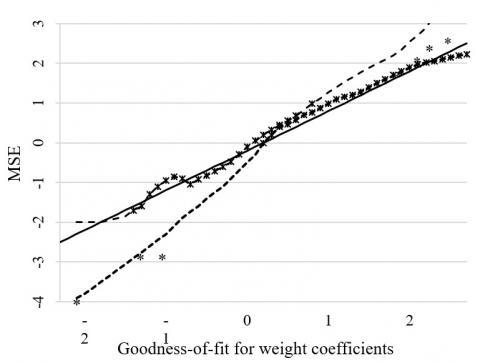Figure 4. The results on MSE

## 5.jpg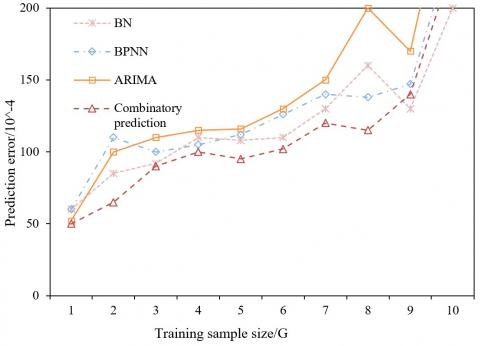Figure 5. Prediction results with 50 training samples

## 6.jpgFigure 6. Prediction results with 100 training samples

Figure 4 compares the relationship between the goodness of fit for the weight coefficients and the mean squared error (MSE) under the above settings and those for uniformly assigned weight coefficients. Obviously, the reasonable allocation of the weight coefficients narrows the gap between prediction error and the actual error, leading to an ideal prediction effect.

To demonstrates its prediction effect, the proposed combinatory prediction model was compared with BN, BPNN and ARIMA. Figures 5 and 6 provide the relationship between sample size and prediction error of the four methods, when there were 50 and 100 training samples, respectively. It can be seen that the combinatory prediction model achieved the smallest prediction error, followed by the BN. By contrast, the ARIMA and BPNN had relatively large prediction errors.

6. Conclusions

This paper discusses the application mechanism of big data technology in the financial field, and clarifies the procedure of preprocessing financial data. Based on the user portrait of financial behaviors, a financial BDA model was designed based on the ML. The algorithm of the proposed model has many advantages: Firstly, the model inherits the merits of three networks, namely, BN, BPNN and ARIMA, in the prediction of massive financial data, by assigning reasonable weighting coefficients to the three networks. Secondly, the parameters of the ML module were optimized through dissimilarity calculation, mitigating the impact of high-dimensional data on the prediction results. Finally, the global convergence ability was enhanced through the reasonable allocation of weight coefficients, such that the prediction would not focus on a single index. Differential experiments show that the proposed user portrait greatly facilitates the construction of causality in a large sample set, and the prediction curve of the proposed combinatory prediction model achieves the smallest prediction error.

Acknowledgment

This work is supported by Hebei Province University Smart Finance Application Technology R&D Center (Grant No.: XGZJ2020013).

References

 Akhmetshin, E.M., Vasilev, V.L. (2016). Control as an instrument of management and institution of economic security. Academy of Strategic Management Journal, 15(3): 163-169.

 Habeeb, R.A.A., Nasaruddin, F., Gani, A., Hashem, I.A.T., Ahmed, E., Imran, M. (2019). Real-time big data processing for anomaly detection: A survey. International Journal of Information Management, 45: 289-307. https://doi.org/10.1016/j.ijinfomgt.2018.08.006

 Atlam, H.F., Walters, R.J., Wills, G.B. (2018). Fog computing and the internet of things: A review. Big Data and Cognitive Computing, 2(2): 10. https://doi.org/10.3390/bdcc2020010

 Shih, D.H., Hsu, H.L., Shih, P.Y. (2019). A study of early warning system in volume burst risk assessment of stock with big data platform. In 2019 IEEE 4th International Conference on Cloud Computing and Big Data Analysis (ICCCBDA), pp. 244-248. https://doi.org/10.1109/ICCCBDA.2019.8725738

 Manogaran, G., Vijayakumar, V., Varatharajan, R., Kumar, P.M., Sundarasekar, R., Hsu, C.H. (2018). Machine learning based big data processing framework for cancer diagnosis using hidden Markov model and GM clustering. Wireless Personal Communications, 102(3): 2099-2116. https://doi.org/10.1007/s11277-017-5044-z

 Raturi, R. (2018). Machine learning implementation for identifying fake accounts in social network. International Journal of Pure and Applied Mathematics, 118(20): 4785-4797.

 García-Gil, D., Luengo, J., García, S., Herrera, F. (2019). Enabling smart data: noise filtering in big data classification. Information Sciences, 479: 135-152. https://doi.org/10.1016/j.ins.2018.12.002

 Sapountzi, A., Psannis, K.E. (2018). Social networking data analysis tools & challenges. Future Generation Computer Systems, 86: 893-913. https://doi.org/10.1016/j.future.2016.10.019

 Back, B.H., Ha, I.K. (2019). Comparison of sentiment analysis from large twitter datasets by naïve Bayes and natural language processing methods. Journal of Information and Communication Convergence Engineering, 17(4): 239-245.

 Ma, A. (2018). China has started ranking citizens with a creepy ‘social credit’ system—here’s what you can do wrong, and the embarrassing, demeaning ways they can punish you. Business Insider, 29.

 Harwell, D. (2019). Faked Pelosi videos, slowed to make her appear drunk, spread across social media. The Washington Post, 24.

 Waterson, J. (2019). Facebook refuses to delete fake Pelosi video spread by Trump supporters. The Guardian, 24.

 Shu, K., Sliva, A., Wang, S., Tang, J., Liu, H. (2017). Fake news detection on social media: A data mining perspective. ACM SIGKDD Explorations Newsletter, 19(1): 22-36. https://doi.org/10.1145/3137597.3137600

 Reis, J.C., Correia, A., Murai, F., Veloso, A., Benevenuto, F. (2019). Supervised learning for fake news detection. IEEE Intelligent Systems, 34(2): 76-81. https://doi.org/10.1109/MIS.2019.2899143

 Li, S., Neupane, A., Paul, S., Song, C., Krishnamurthy, S.V., Chowdhury, A.K.R., Swami, A. (2018). Adversarial perturbations against real-time video classification systems. arXiv preprint arXiv:1807.00458. https://doi.org/10.14722/ndss.2019.23202

 Kobie, N. (2019). The complicated truth about China’s social credit system. Wired UK.

 Cummins, M., Rajan, N.S., Hodge, C., Gouripeddi, R. (2016). Patterns and perceptions of asynchronous video discussion in a graduate health sciences course. Journal of Nursing Education, 55(12): 706-710. https://doi.org/10.3928/01484834-20161114-08

 French, S., Kelly, P. (2017). Visions for Australian tertiary education. R.H. James (Ed.). University of Melbourne.

 Kuzilek, J., Hlosta, M., Zdrahal, Z. (2017). Open university learning analytics dataset. Scientific Data, 4: 170171. https://doi.org/10.1038/sdata.2017.171

 Robinson, I., Webber, J., Eifrem, E. (2015). Graph databases: new opportunities for connected data. O'Reilly Media, Inc.

 Lu, M.F., Xu, B.H. (2018). Influencing factors and prevention of systemic financial risk. People, 6: 43-44.

 Li, X.L. (2017). Research on the risk and prevention of internet finance. Times Finance, (6): 30-31.

 He, P.Y. (2017). Research on application mode and value of big data based on Internet finance. China's Circulation Economy, 31(5): 39-46. https://doi.org/10.14089/j.cnki.cn11-3664/f.2017.05.005

 Dhayne, H., Haque, R., Kilany, R., Taher, Y. (2019). In search of big medical data integration solutions-a comprehensive survey. IEEE Access, 7: 91265-91290. https://doi.org/10.1109/ACCESS.2019.2927491

 Tsang, G., Xie, X., Zhou, S.M. (2019). Harnessing the power of machine learning in dementia informatics research: issues, opportunities, and challenges. IEEE Reviews in Biomedical Engineering, 13: 113-129. https://doi.org/10.1109/RBME.2019.2904488